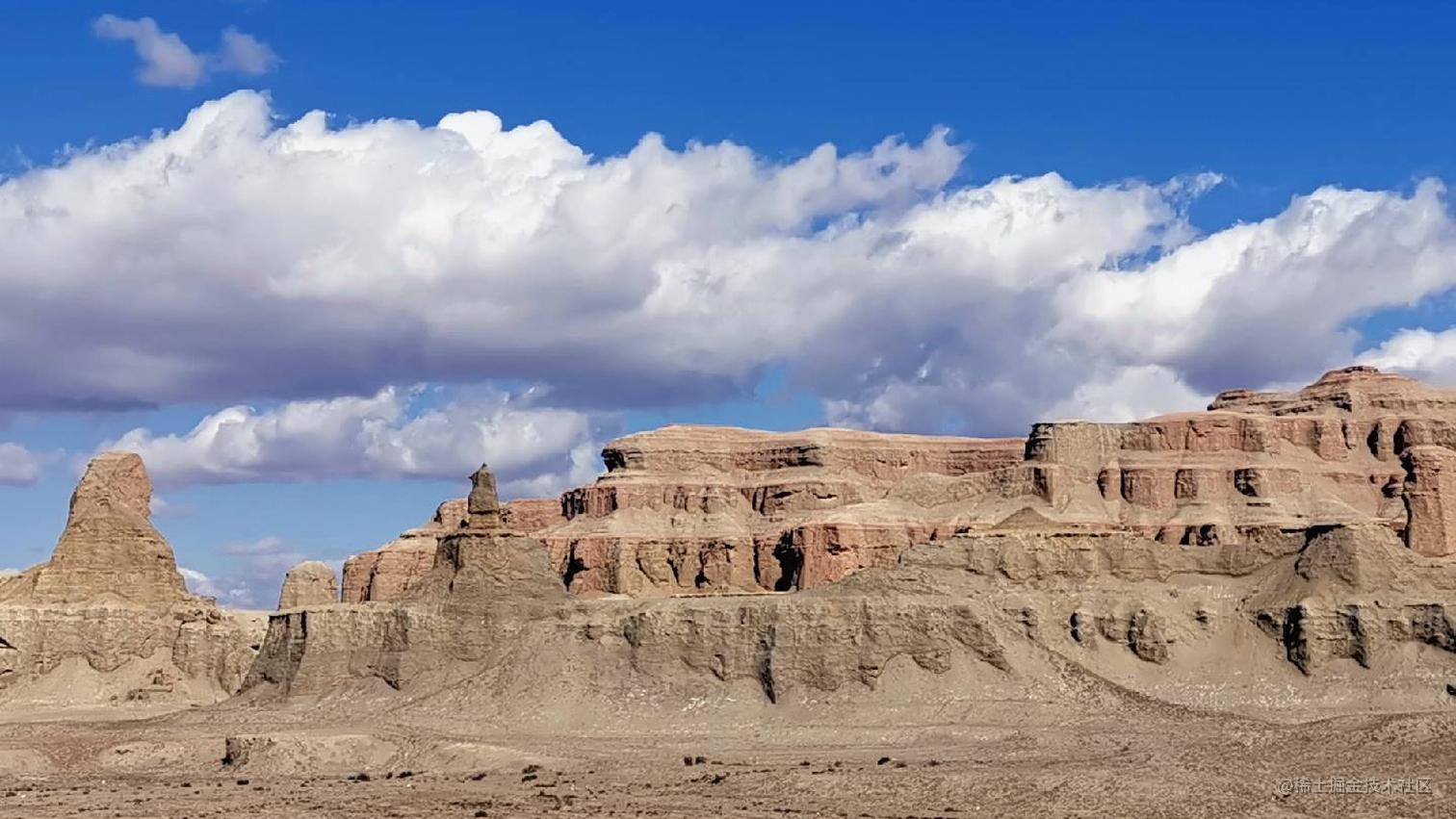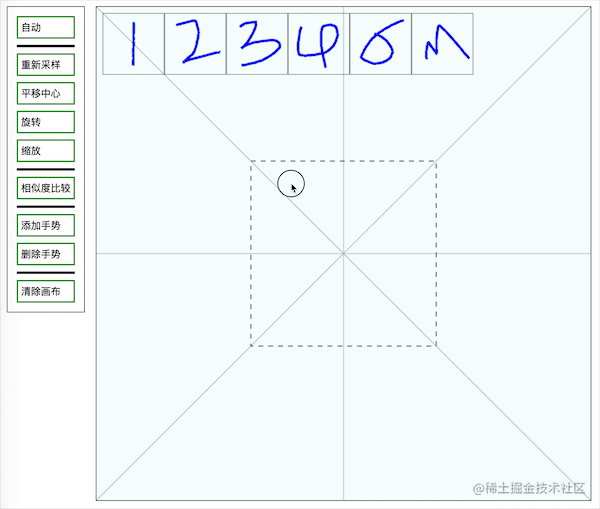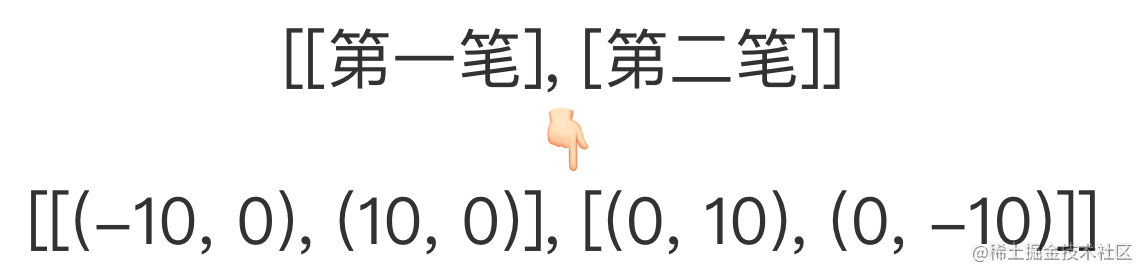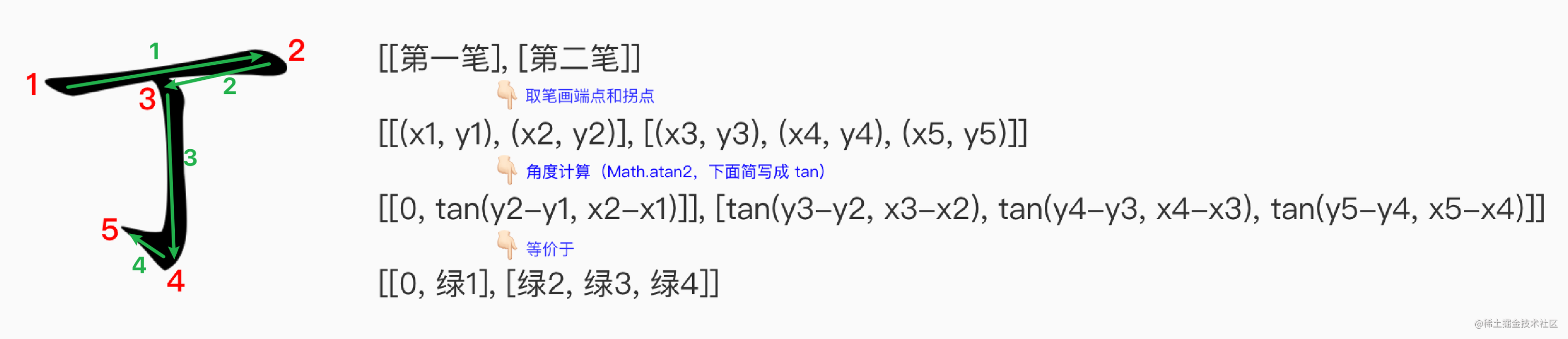# 用 canvas 搞一个手势识别？醍醐灌顶 🤯## 前言如果你是初次看过肯定会觉得很有意思🤩。哈哈，话不多说，让我们直接开撸吧。

## 具体步骤

### 第一步：手势绘制

``````handleMousemove(e: MouseEvent) {
if (!this.isMove) return;
const curPoint = this.getCanvasPos(e);
const lastPoint = this.inputPoints[this.inputPoints.length - 1];
// 画线段
CanvasUtils.drawLine(this.ctx2d, lastPoint, lastPoint, curPoint, curPoint, 'blue', 3);
// 画坐标点
CanvasUtils.drawCircle(this.ctx2d, curPoint, curPoint, 5);
// 如果觉得原始点的数量太多，可以节流
this.inputPoints.push(curPoint);
}

### 第二步：重新取样

``````export type Point = [number, number];
static resample(inputPoints: Point[], sampleCount: number): Point[] {
const len = GeoUtils.getLength(inputPoints);
const unit = len / (sampleCount - 1);
const outputPoints: Point[] = [[...inputPoints]];

let curLen = 0;
let prevPoint = inputPoints;

for (let i = 1; i < inputPoints.length; i++) {
const curPoint = inputPoints[i];
let dx = curPoint - prevPoint;
let dy = curPoint - prevPoint;
let tempLen = GeoUtils.getLength([prevPoint, curPoint]);

while (curLen + tempLen >= unit) {
const ds = unit - curLen;
const ratio = ds / tempLen;
const newPoint: Point = [prevPoint + dx * ratio, prevPoint + dy * ratio];
outputPoints.push(newPoint);

curLen = 0;
prevPoint = newPoint;
dx = curPoint - prevPoint;
dy = curPoint - prevPoint;
tempLen = GeoUtils.getLength([prevPoint, curPoint]);
}
prevPoint = curPoint;
curLen += tempLen;
}
while (outputPoints.length < sampleCount) {
outputPoints.push([...prevPoint]);
}
return outputPoints;
}

### 第二步：平移

``````// 对每个坐标点进行平移
static translate(points: Point[], dx: number, dy: number) {
points.forEach((p) => {
p += dx;
p += dy;
});
}

### 第三步：旋转

``````// 计算需要旋转到最近辅助线的弧度，center 为中心点，startPoint 为手势起始点，sublineCount 为坐标等分数量
static computeRadianToSubline(center: Point, startPoint: Point, sublineCount: number): number {
const dy = startPoint - center;
const dx = startPoint - center;

const unitRadian = TWO_PI / sublineCount;
}
// 对每个坐标点进行旋转
static rotate(points: Point[], radian: number) {
points.forEach((p) => {
let [x, y] = p;
p = cos * x - sin * y;
p = sin * x + cos * y;
});
}

### 第四步：缩放

``````// 再次提醒下因为我们已经把坐标系移到了画布中央，画布中心和手势中心是重合的，所以直接乘以缩放倍速就可以了
static scale(points: Point[], scale: number) {
points.forEach((p) => {
let [x, y] = p;
p = x * scale;
p = y * scale;
});
}

### 第五步：手势录入

• 缩略图：动态地创建一个 canvas 来绘制手势，再通过 drawImage 绘制到画布上，这个其实和第一步是一样的，只不过图变小了。用原始点或采样点画都可以（原始点比较精确），毕竟是缩略图，看不出来太大差别。
• 保存数据：采样坐标点肯定是要保存的，毕竟我们辛辛苦苦标准化了这么久，其它的想保存啥就保存啥。

### 第六步：比较（重点）

``````static squaredEuclideanDistance(points1, points2) {
let squaredDistance = 0;
const count = points1.length;
for (let i = 0; i < count; i++) {
const p1 = points1[i];
const p2 = points2[i];
const dx = p1 - p2;
const dy = p1 - p2;
squaredDistance += dx * dx + dy * dy;
}
return squaredDistance;
}

``````// 计算余弦相似度
static calcCosDistance(vector1: number[], vector2: number[]): number {
let similarity = 0;
vector1.forEach((v1, i) => {
const v2 = vector2[i];
similarity += v1 * v2;
});
return similarity; // 相似度介于 -1~1
}

1. 想办法把原始数据转换成长度相同的一维数组`[a, b, c, ..., n]`（虽然是一维数组，但是是 n 维向量，不理解没关系）
2. 遍历现有数据，分别求出对应的余弦值，找出相似度值最高的那一个。

## 注意事项

• 手势具有方向性：我们可以识别`|``/`，因为他们经过旋转都靠近`y`轴，但是`|``一`就不行了，一个是 y 轴一个是 x 轴。所以如果我们要想把`|``一`识别成一个东西可以这样子搞，把`|`多旋转几个角度，在每个角度都判断一下是否相似。
• 手势的宽高比会影响结果：比如你画一个正方形和一个长长扁扁的矩形是不相似的。
• 采样点的数量：过多过少都不行，过多效率低，对图形一致性要求也高，反之同理。
• 手势的复杂度：图形的识别率和图形的复杂性没有太大关系。简单的图形由于特征不明显，容易出错，比如多边型和圆。复杂的图形，采样点就容易被稀释，得到的特征比较粗。
• 应用场景：大家可以自己想想这个东西除了用在手势还能用在那里？这里举个例子，比如数学老师在远程上课、写板书的时候，经常需要徒手画圆或者画正方形，这里我们就可以帮其自动校正，如果画的像一个圆就自动重新生成一个正圆，也许描述的比较苍白，所以大家可以自行脑补一下画面😂。

## 比较的基本套路（可跳过）

• 特征提取（就是处理数据的过程）：不管是什么东西，都有对应的原始数据，我们要做的就是将其（经过层层处理）转换成同一个框架维度下（也就是标准化），通常就是将原始数据转换成长度相同的一维数组（再次强调虽然是一维数组，但其实是 n 维向量）。
• 算法识别（就是比较数据的过程）：通过某种算法（比如上面提到的欧氏距离和余弦相似度）进行逐一对比。类似的还有网格识别（先把图片马赛克化，像素粒度就变粗了，然后根据像素颜色差值进行比较，这个方法是适用于以缩略图找原图）、方向识别（比如只要手势顺序是先向右再向下再向左再向上就认为是矩形）等。 显然不同的特征和算法就造成了结果的千差万别（效率啊、准确率等，还有薪资待遇🤨？），优化的手段也是百花齐放，所以也就没有通用的算法，只有适合的算法，因地制宜。我们以一个极其简单的推荐算法为例，推荐算法的问题在某种程度上可以转换成两个人的喜好相似程度：
喜好干饭摸鱼睡觉就是玩...
甲（咸鱼）...
乙（翻身）...
??...

## 关于多笔画（可跳过）

• 提取每个汉字的笔画特征，一般可以采集起始点、终点和中间的转折点。数据大概长下面这个样子：• 处理数据（标准化的过程，比如把每个字移到画布中心，缩放成一样的大小）
• 比较数据（选个算法，这里就是先判断下笔画数，再简单的将单笔相似度相加求和） 这就完了？当然还差得远呢，问题一抓一大把。比如：
• 由于存在连笔的情况，一笔可能写成两笔，所以我们应该允许笔画的误差在 2 左右，但是在最终排序时，笔画数越接近的，优先级越高。
• 每一笔当中至少包含起点和终点，中间可能有几个拐点，如果比较的时候单笔的坐标点数量不同该怎么处理？一种方式是进行插值计算，另一种方式是取最初的采样点信息。
• 采用上述的方式如果我写了个`丁`字是不是好像也能识别出来，大体都是一横一竖，有没有什么办法可以避免呢？当然是有的，现在我们每一笔保存的不再是点的坐标，而是该点与前一个点连线的角度，如果是每一笔的起始点，就拿上一笔的终点作为前一个点，说起来比较抽象，所以我又画了张图👇🏻（很简单的一张图，不要被吓到😂）：大家想想如果是`十`字，在上图的第二个角度（绿2）中是不是就可以明显区分开了。另外我们只保存了两两点之间的角度，还省了不少空间呢。
看起来好像没问题了？不，还是差得远呢。你想想要是笔画顺序不对咋整。还是以`十`为例，我先写竖再写横咋整。啊这。。。其实还有其他识别方法，比如把文字按坐标轴切分成四块，分四段校验，这就不深入了，点到即止（毕竟就懂点皮毛）。